### How To Master Techniques Of Proof For AS Maths

Proof questions at AS Level require you to apply simple mathematical rules to show that certain statements are universally true (or untrue).

They are almost like logic puzzles for mathematicians, so they can be quite fun (honestly)!

Once you’ve become familiar with the ways that examiners phrase the questions and you’ve learnt the right notation to use, proof questions can be a piece of cake.

Read on to find everything you need to know about AS Proof questions, straight from the brain of a Save My Exams Maths teacher. Remember, if you’re looking to get ahead of the class, don’t skip over the worked examples

This blog post has been adapted from our new teacher-written OCR AS Maths: Pure Revision Notes collection.#### Notation in Proof

The below diagram provides a helpful guide to the notation which you should use when ‘proving’. Don’t try to invent your own – the examiners won’t give you any marks!Remember – the term integer refers to a whole number. A set of integers is a group of whole numbers.

#### Proof by Exhaustion

This method literally requires you to ‘exhaust’ the possibilities – i.e. check all values – when proving something to be true. It’s important to only use this technique when it’s sensible to do so – don’t be testing tens (or hundreds) or values!

An example:

The set of numbers S is defined as all positive integers greater than 5 and less than 10. Prove by exhaustion that the square of all values in S differ from a multiple of 5 by 1.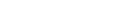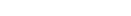#### Proof by Counter Example

To prove that a statement is untrue, you just need to find one exception to the rule.

You might also be asked to disprove a statement – but the same method applies.

You’ll need to get your detective hat on to find the anomaly! The below diagram provides some useful tips for finding this value…An example:

Use a counter example to prove that the difference between any two square numbers is not always odd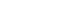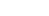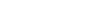(an EVEN number, proving the statement)

Right, that’s the theory covered. Let’s go deeper into this topic with three worked examples. Give them a try for yourself before looking at the answers!#### Question 1

Prove that the sum of any three consecutive even numbers is always a multiple of 2, but not always a multiple of 4.

Three even consecutive numbers =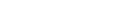Their sum =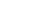Factorised =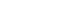– Which is always a multiple of 2, but not always a multiple of 4!

#### Question 2

Prove that 23 is a prime number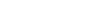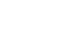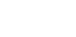There is a rule that all numbers can be written as a product of their prime factors. So, all non-primes should have at least one other factor (besides themselves and 1).

Because 23 does not have any prime factors below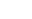, we have proven it is a prime number.

#### Question 3

Prove that, if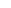is odd,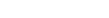is odd, and that ifis even,is even

First, factorise the equation to get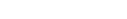Ifis odd,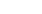andare also odd.

The product of three odd numbers is always odd

Ifis even,andare also even.

The product of three even numbers is always even

Are you ready to try some more practice questions? Head on over to our OCR AS Maths: Pure Topic Questions! Don’t worry if you’re not feeling 100% confident yet; every question is accompanied by a full answer explanation.

If you’d like to revise another topic, check out the full Revision Notes collection here